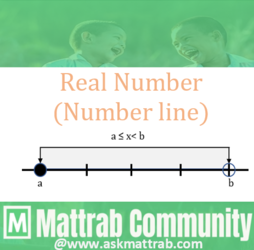Real Numbers - Number LineHello Viewers!!
Before understanding the number line, please visit
this note for the basics.

Inequality﻿
An inequality is a relation which shows the comparison between two non-equal numbers or expressions using some mathematical signs.
Mathematical signs used in Inequality:
i.     :    "less than or equal to"      → x-3≤7 {if x≤10}
ii.    <:    "less than"                          → x-3<7 {if x<10}
iii.   ≠:    "not equal to"                     → 2≠3
iv.   >:    "greater than"                     → 7>4
v.    :    "greater than or equal to" → x+3≥7 {if x≥4}

﻿Interval
﻿The set of points on the real number line which gives range of real values between two numbers that may or may not include the two end point numbers is called interval. The interval is enclosed by '[  ]' or '( )' or both.

﻿Types of Interval
Let {a, b} ∈ R such that a<b
1. Open Interval
→The interval which doesn't include the end points 'a' and 'b'.
→denoted as: (a, b)
→Symbolically, (a, b)={x: a<x<b}
→Number Line:2. Close Interval
→The interval which includes end points 'a' and 'b'.
→denoted as: [a, b]
→Symbolically, [a, b]={x: a ≤x ≤b}
→Number Line:3. Left Open Interval/Right Close Interval
→The interval that doesn't include the smaller end point 'a' but includes larger end point 'b'.
→denoted as: (a, b]             {∵ it is open in left and open interval is denoted by '(  )'.}
→Symbolically, (a, b]={x: a<x≤ b}
→Number Line:4. Right Open Interval/Left Close Interval
→The interval that includes the smaller end point 'a' but doesn't include larger end point 'b'.
→denoted as: [a, b)             {∵ it is open in right and open interval is denoted by '(  )'.}
→Symbolically, [a, b)={x: a≤ x< b}
→Number Line: